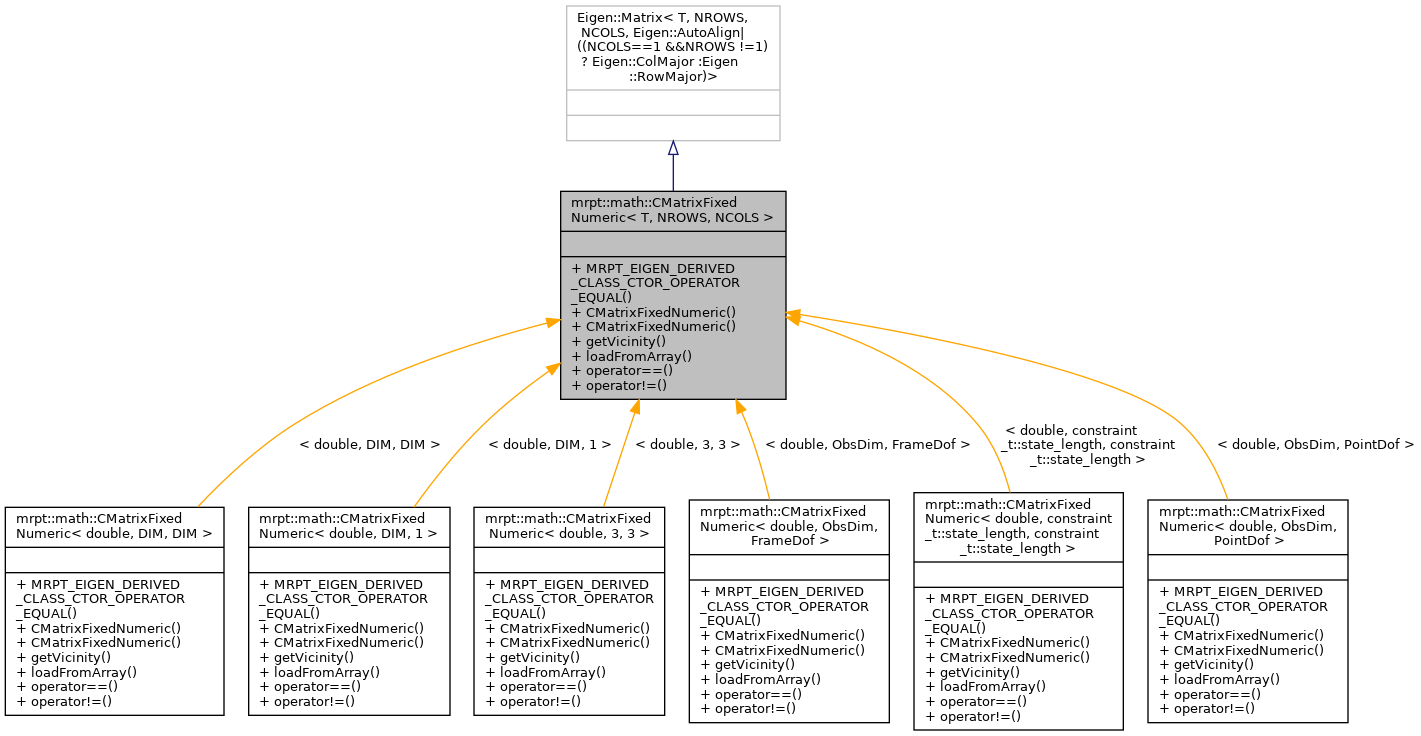MRPT  1.9.9
mrpt::math::CMatrixFixedNumeric< T, NROWS, NCOLS > Class Template Reference

## Detailed Description

### template<typename T, size_t NROWS, size_t NCOLS> class mrpt::math::CMatrixFixedNumeric< T, NROWS, NCOLS >

A numeric matrix of compile-time fixed size.

Basically, this class is a wrapper on Eigen::Matrix<T,NROWS,NCOLS>, but with a RowMajor element memory layout (except for column vectors).

These matrices also have iterators to access all the elements in the matrix as a sequence, starting from the element (0,0), then row by row, from left to right.

Note
This class exists for backward compatibility of ancient times when MRPT didn't rely on Eigen, feel free to directly use Eigen::Matrix<> types instead.
CMatrixTemplateNumeric (for dynamic-size matrices)
Note
For a complete introduction to Matrices and vectors in MRPT, see: http://www.mrpt.org/Matrices_vectors_arrays_and_Linear_Algebra_MRPT_and_Eigen_classes

Definition at line 40 of file CMatrixFixedNumeric.h.

`#include <mrpt/math/CMatrixFixedNumeric.h>`

Inheritance diagram for mrpt::math::CMatrixFixedNumeric< T, NROWS, NCOLS >:## Public Types

using Base = Eigen::Matrix< T, NROWS, NCOLS, Eigen::AutoAlign|((NCOLS==1 &&NROWS !=1) ? Eigen::ColMajor :Eigen::RowMajor)>

using mrpt_autotype = CMatrixFixedNumeric< T, NROWS, NCOLS >

## Public Member Functions

MRPT_EIGEN_DERIVED_CLASS_CTOR_OPERATOR_EQUAL (CMatrixFixedNumeric) inline CMatrixFixedNumeric()
Default constructor, initializes all elements to zero. More...

CMatrixFixedNumeric (const T *vals)
Constructor from an array in row major. More...

CMatrixFixedNumeric (TConstructorFlags_Matrices)
Constructor which leaves the matrix uninitialized. More...

template<size_t N, typename ReturnType >
ReturnType getVicinity (size_t c, size_t r) const

template<typename Derived >
bool operator== (const Eigen::MatrixBase< Derived > &m2) const
== comparison of two matrices; it differs from default Eigen operator in that returns false if matrices are of different sizes instead of raising an assert. More...

template<typename Derived >
bool operator!= (const Eigen::MatrixBase< Derived > &m2) const
!= comparison of two matrices; it differs from default Eigen operator in that returns true if matrices are of different sizes instead of raising an assert. More...

## ◆ Base

template<typename T, size_t NROWS, size_t NCOLS>
 using mrpt::math::CMatrixFixedNumeric< T, NROWS, NCOLS >::Base = Eigen::Matrix< T, NROWS, NCOLS, Eigen::AutoAlign | ((NCOLS == 1 && NROWS != 1) ? Eigen::ColMajor : Eigen::RowMajor)>

Definition at line 52 of file CMatrixFixedNumeric.h.

## ◆ mrpt_autotype

template<typename T, size_t NROWS, size_t NCOLS>
 using mrpt::math::CMatrixFixedNumeric< T, NROWS, NCOLS >::mrpt_autotype = CMatrixFixedNumeric

Definition at line 53 of file CMatrixFixedNumeric.h.

## ◆ CMatrixFixedNumeric() [1/2]

template<typename T, size_t NROWS, size_t NCOLS>
 mrpt::math::CMatrixFixedNumeric< T, NROWS, NCOLS >::CMatrixFixedNumeric ( const T * vals )
inline

Constructor from an array in row major.

Definition at line 62 of file CMatrixFixedNumeric.h.

## ◆ CMatrixFixedNumeric() [2/2]

template<typename T, size_t NROWS, size_t NCOLS>
 mrpt::math::CMatrixFixedNumeric< T, NROWS, NCOLS >::CMatrixFixedNumeric ( TConstructorFlags_Matrices )
inline

Constructor which leaves the matrix uninitialized.

Example of usage: CMatrixFixedNumeric<double,3,2> M(mrpt::math::UNINITIALIZED_MATRIX);

Definition at line 67 of file CMatrixFixedNumeric.h.

## ◆ getVicinity()

template<typename T, size_t NROWS, size_t NCOLS>
template<size_t N, typename ReturnType >
 ReturnType mrpt::math::CMatrixFixedNumeric< T, NROWS, NCOLS >::getVicinity ( size_t c, size_t r ) const
inline

Definition at line 69 of file CMatrixFixedNumeric.h.

template<typename T, size_t NROWS, size_t NCOLS>
 void mrpt::math::CMatrixFixedNumeric< T, NROWS, NCOLS >::loadFromArray ( const T * vals )
inline

## ◆ MRPT_EIGEN_DERIVED_CLASS_CTOR_OPERATOR_EQUAL()

template<typename T, size_t NROWS, size_t NCOLS>
 mrpt::math::CMatrixFixedNumeric< T, NROWS, NCOLS >::MRPT_EIGEN_DERIVED_CLASS_CTOR_OPERATOR_EQUAL ( CMatrixFixedNumeric< T, NROWS, NCOLS > )
inline

Default constructor, initializes all elements to zero.

Definition at line 55 of file CMatrixFixedNumeric.h.

## ◆ operator!=()

template<typename T, size_t NROWS, size_t NCOLS>
template<typename Derived >
 bool mrpt::math::CMatrixFixedNumeric< T, NROWS, NCOLS >::operator!= ( const Eigen::MatrixBase< Derived > & m2 ) const
inline

!= comparison of two matrices; it differs from default Eigen operator in that returns true if matrices are of different sizes instead of raising an assert.

Definition at line 95 of file CMatrixFixedNumeric.h.

## ◆ operator==()

template<typename T, size_t NROWS, size_t NCOLS>
template<typename Derived >
 bool mrpt::math::CMatrixFixedNumeric< T, NROWS, NCOLS >::operator== ( const Eigen::MatrixBase< Derived > & m2 ) const
inline

== comparison of two matrices; it differs from default Eigen operator in that returns false if matrices are of different sizes instead of raising an assert.

Definition at line 86 of file CMatrixFixedNumeric.h.

 Page generated by Doxygen 1.8.14 for MRPT 1.9.9 Git: 7d5e6d718 Fri Aug 24 01:51:28 2018 +0200 at lun nov 2 08:35:50 CET 2020